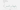Back to all

## Accurate Uncertainties for Deep Learning Using Calibrated Regression

### \\ 03.01.2019We recently published some novel ML research at ICML, one of the leading conferences in machine learning. Below follows the abstract and intro from our paper.

#### ABSTRACT

Methods for reasoning under uncertainty are a key building block of accurate and reliable machine learning systems. Bayesian methods provide a general framework to quantify uncertainty. However, because of model misspecification and the use of approximate inference, Bayesian uncertainty estimates are often inaccurate — for example, a 90% credible interval may not contain the true outcome 90% of the time. Here, we propose a simple procedure for calibrating any regression algorithm; when applied to Bayesian and probabilistic models, it is guaranteed to produce calibrated uncertainty estimates given enough data. Our procedure is inspired by Platt scaling and extends previous work on classification. We evaluate this approach on Bayesian linear regression, feedforward, and recurrent neural networks, and find that it consistently outputs well-calibrated credible intervals while improving performance on time series forecasting and model-based reinforcement learning tasks.

#### 1. INTRODUCTION

Methods for reasoning and making decisions under uncertainty are an important building block of accurate, reliable, and interpretable machine learning systems. In many applications — ranging from supply chain planning to medical diagnosis to autonomous driving — faithfully assessing uncertainty can be as important as obtaining high accuracy. This paper explores uncertainty estimation over continuous variables in the context of modern deep learning models. Bayesian approaches provide a general framework for dealing with uncertainty (Gal, 2016). type: embedded-entry-inline id: 1U0cif6enXb5eDkDpE34Vx Bayesian methods define a probability distribution over model parameters and derive uncertainty estimates by intergrating over all possible model weights. Recent advances in variational inference have greatly increased the scalability and usefulness of these approaches (Blundell et al., 2015). type: embedded-entry-inline id: 1U0cif6enXb5eDkDpE34Vx

Here, we propose a new procedure for recalibrating any regression algorithm that is inspired by Platt scaling for classification. When applied to Bayesian and probabilistic deep learning models, it always produces calibrated credible intervals given a sufficient amount of i.i.d. data.

We evaluate our proposed algorithm on a range of Bayesian models, including Bayesian linear regression as well as feedforward and recurrent Bayesian neural networks. Our method consistently produces well-calibrated confidence estimates, which are in turn useful for several tasks in time series forecasting and model-based reinforcement learning.

Contributions In summary, we introduce a simple technique for recalibrating the output of any regression algorithm, extending recalibration methods such as Platt scaling that were previously applicable only to classification. We then use this technique to solve an important problem in Bayesian deep learning: the miscalibration of credible intervals. We show that our results are useful in time series forecasting and in model-based reinforcement learning.

#### 2. CALIBRATED CLASSIFICATION

This section is a concise overview of calibrated classification (Platt, 1999), and offers a reinterpretation of existing techniques that will be useful for deriving an extension to the regression and Bayesian settings in the next section.

Notation. We are given a labeled dataset xt, yt ∈ X × Y for t = 1, 2, ..., T of i.i.d. realizations of random variables X, Y ∼ P, where P is the data distribution. Given xt, a forecaster H : X → (Y → [0, 1]) outputs a probability distribution Ft(y) targeting the label yt. When Y is continuous, Ft is a cumulative probability distribution (CDF). In this section, we assume for simplicity that Y = {0, 1}.

#### References

Yarin Gal Uncertainty in Deep Learning. PhD thesis, University of Cambridge, 2016.

Charles Blundell, Julien Cornebise, Koray Kavukcuoglu, and Daan Wierstra Weight uncertainty in neural networks. arXiv preprint arXiv:1505.05424, 2015.

Balaji Lakshminarayanan, Alexander Pritzel, and Charles Blundell Simple and scalable predictive uncertainty estimation using deep ensembles. arXiv preprint arXiv:1612.01474, 2017.

Yarin Gal, Jiri Hron, and Alex Kendall Uncertainty in Deep Learning. PhD thesis, University of Cambridge, 2016.